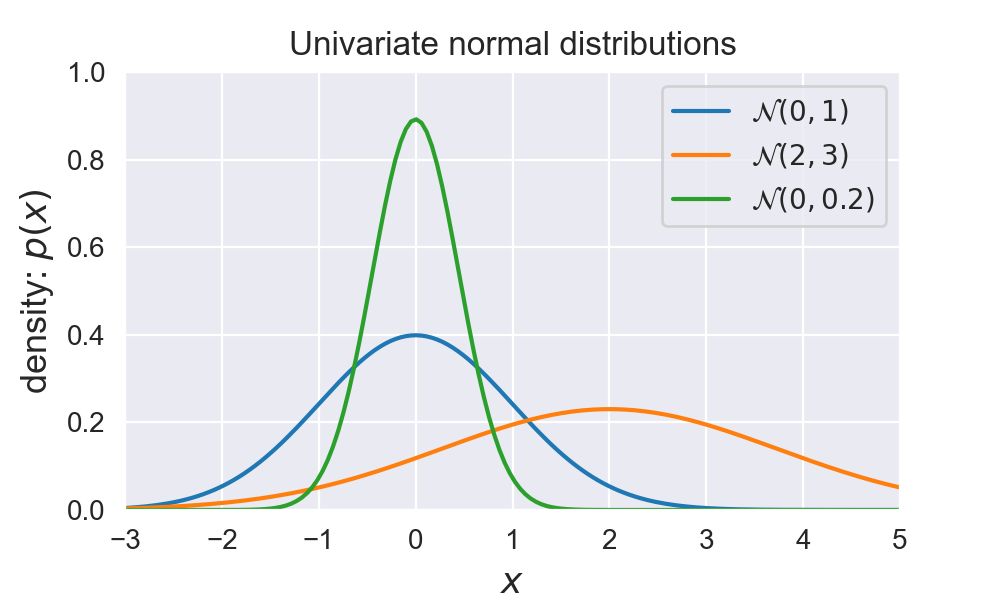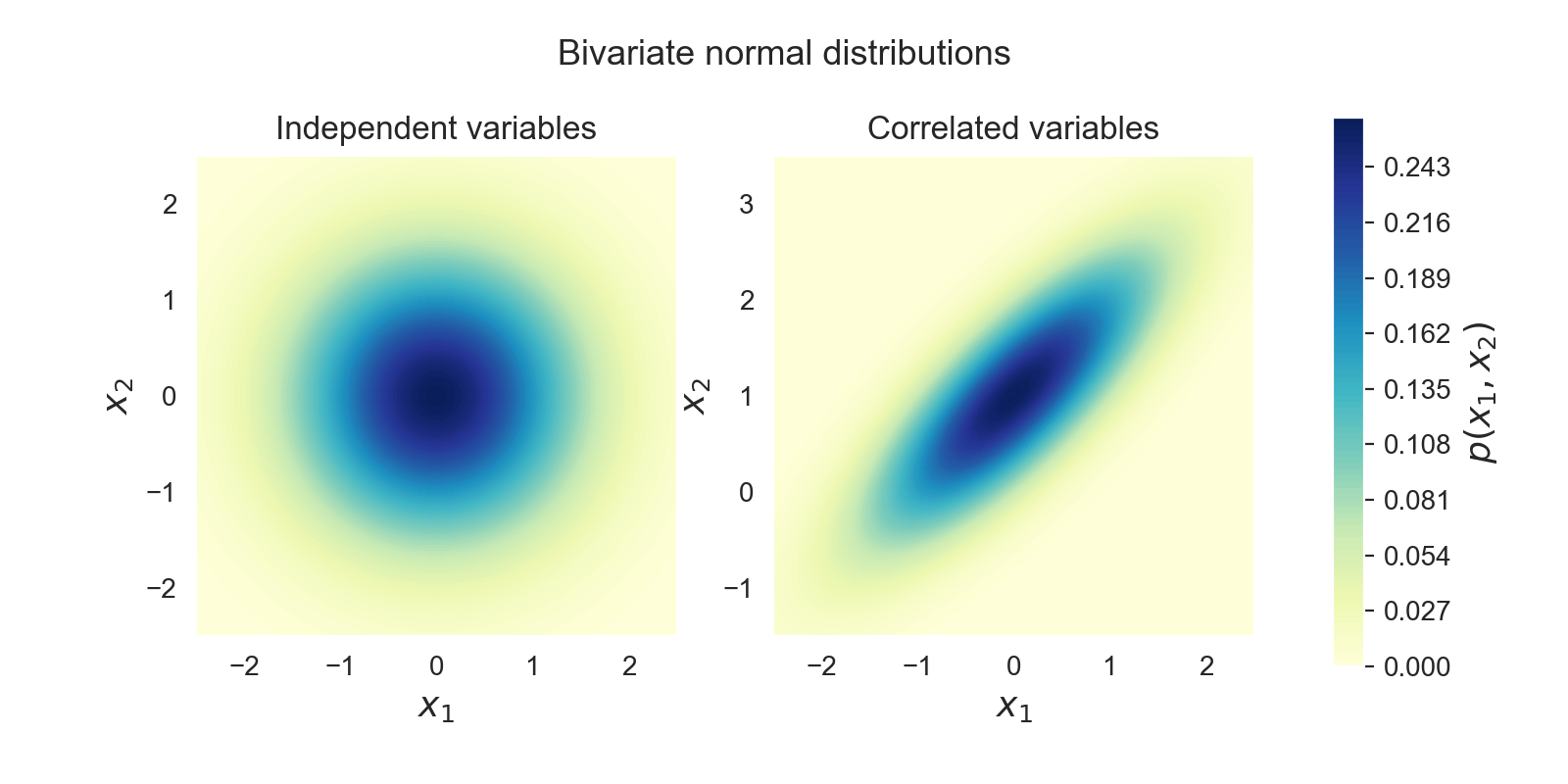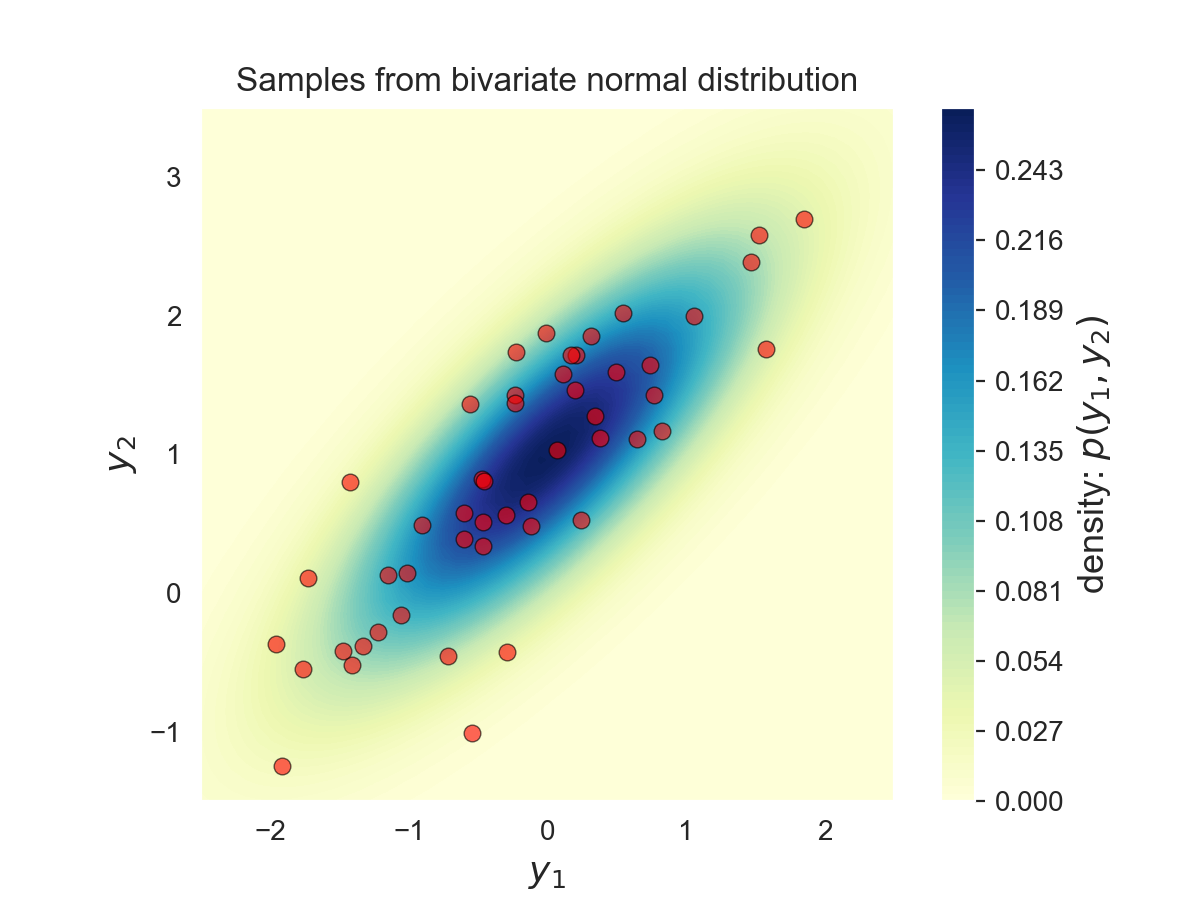# Multivariate normal distribution

This post will introduce the multivariate normal (multivariate Gaussian) distribution. It illustrates how to represent, visualize, sample, and compute conditionals and marginals from this distribution.

This post assumes a basic understanding of probability theory, probability distributions and linear algebra.

In :

## Univariate normal distribution ¶

The normal distribution , also known as the Gaussian distribution, is so called because its based on the Gaussian function . This distribution is defined by two parameters: the mean $\mu$, which is the expected value of the distribution, and the standard deviation $\sigma$, which corresponds to the expected deviation from the mean. The square of the standard deviation is typically referred to as the variance $\sigma^2$. We denote this distribution as:

$$\mathcal{N}(\mu, \sigma^2)$$

Given this mean and variance we can calculate the probility densitiy function (pdf) of the normal distribution with the normalised Gaussian function. For a value $x$ the density is:

$$p(x \mid \mu, \sigma) = \frac{1}{\sqrt{2\pi\sigma^2}} \exp{ \left( -\frac{(x - \mu)^2}{2\sigma^2}\right)}$$

We call this distribution the univariate normal because it consists of only one random normal variable. Three examples of univariate normal distributions with different mean and variance are plotted in the next figure:

In :
def univariate_normal(x, mean, variance):
"""pdf of the univariate normal distribution."""
return ((1. / np.sqrt(2 * np.pi * variance)) *
np.exp(-(x - mean)**2 / (2 * variance)))

In :## Multivariate normal distribution ¶

The multivariate normal distribution is a multidimensional generalisation of the one-dimensional normal distribution . It represents the distribution of a multivariate random variable that is made up of multiple random variables that can be correlated with eachother.

Like the normal distribution, the multivariate normal is defined by sets of parameters: the mean vector $\mathbf{\mu}$, which is the expected value of the distribution; and the covariance matrix $\Sigma$, which measures how dependend two random variables are and how they change together. We denote the covariance between variable $X$ and $Y$ as $C(X,Y)$.

The multivariate normal with dimensionality $d$ has a joint probability density given by:

$$p(\mathbf{x} \mid \mathbf{\mu}, \Sigma) = \frac{1}{\sqrt{(2\pi)^d \lvert\Sigma\rvert}} \exp{ \left( -\frac{1}{2}(\mathbf{x} - \mathbf{\mu})^T \Sigma^{-1} (\mathbf{x} - \mathbf{\mu}) \right)}$$

Where $\mathbf{x}$ a random vector of size $d$, $\mathbf{\mu}$ is the mean vector, $\Sigma$ is the ( symmetric , positive definite ) covariance matrix (of size $d \times d$), and $\lvert\Sigma\rvert$ its determinant . We denote this multivariate normal distribution as:

$$\mathcal{N}(\mathbf{\mu}, \Sigma)$$
In :
def multivariate_normal(x, d, mean, covariance):
"""pdf of the multivariate normal distribution."""
x_m = x - mean
return (1. / (np.sqrt((2 * np.pi)**d * np.linalg.det(covariance))) *
np.exp(-(np.linalg.solve(covariance, x_m).T.dot(x_m)) / 2))


Examples of two bivariate normal distributions are plotted below.

The figure on the left is a bivariate distribution with the covariance between $x_1$ and $x_2$ set to $0$ so that these 2 variables are independent:

$$\mathcal{N}\left( \begin{bmatrix} 0 \\ 0 \end{bmatrix}, \begin{bmatrix} 1 & 0 \\ 0 & 1 \end{bmatrix}\right)$$

The figure on the right is a bivariate distribution with the covariance between $x_1$ and $x_2$ set to be different than $0$ so that both variables are correlated. Increasing $x_1$ will increase the probability that $x_2$ will also increase:

$$\mathcal{N}\left( \begin{bmatrix} 0 \\ 1 \end{bmatrix}, \begin{bmatrix} 1 & 0.8 \\ 0.8 & 1 \end{bmatrix}\right)$$
In :### Affine transformations of the multivariate normal ¶

It is possible to transform a multivariate normal distribution into a new normal distribution with an affine transformation . More specificaly if $X$ is normally distributed and $Y = LX + u$ with $L$ a linear transformation and $u$ a vector then $Y$ is also normally distributed with mean $\mu_{Y} = u + L\mu_{X}$ and covariance matrix $\Sigma_{Y} = L\Sigma_{X}L^T$.

$$Y \sim \mathcal{N}(\mu_{Y}, \Sigma_{Y}) \quad\quad X \sim \mathcal{N}(\mu_{X}, \Sigma_{X}) \\ \mathcal{N}(\mu_{Y}, \Sigma_{Y}) = \mathcal{N}(u + L\mu_{X}, L\Sigma_{X}L^T) = L\mathcal{N}(\mu_{X}, \Sigma_{X}) + u$$

This can be proven als follows:

$$\mu_{Y} = \mathbb{E}[Y] = \mathbb{E}[LX + u] = \mathbb{E}[LX] + u = L\mu_{X} + u$$$$\begin{split} \Sigma_{Y} & = \mathbb{E}[(Y-\mu_{Y})(Y-\mu_{Y})^\top] \\ & = \mathbb{E}[(LX+u - L\mu_{X}-u)(LX+u - L\mu_{X}-u)^\top] \\ & = \mathbb{E}[(L(X-\mu_{X})) (L(X-\mu_{X}))^\top] \\ & = \mathbb{E}[L(X-\mu_{X}) (X-\mu_{X})^\top L^\top] \\ & = L\mathbb{E}[(X-\mu_{X})(X-\mu_{X})^\top]L^\top \\ & = L\Sigma_{X}L^\top \end{split}$$

## Sampling from a multivariate normal ¶

The previous formula helps us to sample from any multivariate Guassian .
To do this sampling we can sample $X$ from the standard normal distribution $X \sim \mathcal{N}(0, I_d)$, where the mean is the vector $\mu=0$ and the covariance is the identity matrix $\Sigma=I_d$. Sampling from this distribution is easier because each variable in $X$ is independent from all other variables, we can just sample each variable separately.

It is then possible to sample $Y$ from $\mathcal{N}(\mu_{Y}, \Sigma_{Y})$ by sampling $X$ and applying the affine transform on the samples. This tranform is $Y = LX + u$ where we know from the previous section that the covariance of $Y$ will be $\Sigma_{Y} = L\Sigma_{X}L^\top$. Since $\Sigma_{X}=I_d$ we can write that $\Sigma_{Y} = L I_d L^\top = L L^\top$. $L$ can now be found by a technique called the Cholesky decompostion which does exactly the decomposition we need. The vector $u$ is then $\mu_{Y}$ since $\mu_{X}=0$ ($u = \mu_{Y} - L\mu_{X}$).

Lets try this out by sampling 50 samples from:

$$Y \sim \mathcal{N}\left( \begin{bmatrix} 0 \\ 1 \end{bmatrix}, \begin{bmatrix} 1 & 0.8 \\ 0.8 & 1 \end{bmatrix}\right)$$

The sampling is done by the following code and the samples are plotted as red dots on the probability density surface below.

In :
# Sample from:
d = 2 # Number of dimensions
mean = np.matrix([[0.], [1.]])
covariance = np.matrix([
[1, 0.8],
[0.8, 1]
])

# Create L
L = np.linalg.cholesky(covariance)
# Sample X from standard normal
n = 50  # Samples to draw
X = np.random.normal(size=(d, n))
# Apply the transformation
Y = L.dot(X) + mean

In :## Marginal and Conditional normal distributions ¶

If both $\mathbf{x}$ and $\mathbf{y}$ are jointly normal random vectors defined as: $$\begin{bmatrix} \mathbf{x} \\ \mathbf{y} \end{bmatrix} \sim \mathcal{N}\left( \begin{bmatrix} \mu_{\mathbf{x}} \\ \mu_{\mathbf{y}} \end{bmatrix}, \begin{bmatrix} A & C \\ C^T & B \end{bmatrix} \right) = \mathcal{N}(\mu, \Sigma) , \qquad \Sigma^{-1} = \Lambda = \begin{bmatrix} \tilde{A} & \tilde{C} \\ \tilde{C}^T & \tilde{B} \end{bmatrix}$$

In :
d = 2  # dimensions
mean = np.matrix([[0.], [1.]])
cov = np.matrix([
[1, 0.8],
[0.8, 1]
])

# Get the mean values from the vector
mean_x = mean[0,0]
mean_y = mean[1,0]
# Get the blocks (single values in this case) from
#  the covariance matrix
A = cov[0, 0]
B = cov[1, 1]
C = cov[0, 1]  # = C transpose in this case


### Marginal distribution ¶

A marginal distribution is the distribution of a subset of random variables from the original distribution. It represents the probabilities or densities of the variables in the subset without reference to the other values in the original distribution.

In our case of the 2D multivariate normal the marginal distibutions are the univariate distributions of each component $\mathbf{x}$ and $\mathbf{y}$ seperately. They are defined as:

$$\begin{split} p(\mathbf{x}) & = \mathcal{N}(\mu_{\mathbf{x}}, A) \\ p(\mathbf{y}) & = \mathcal{N}(\mu_{\mathbf{y}}, B) \end{split}$$
In :